# Physics - Classical Mechanics - Impulse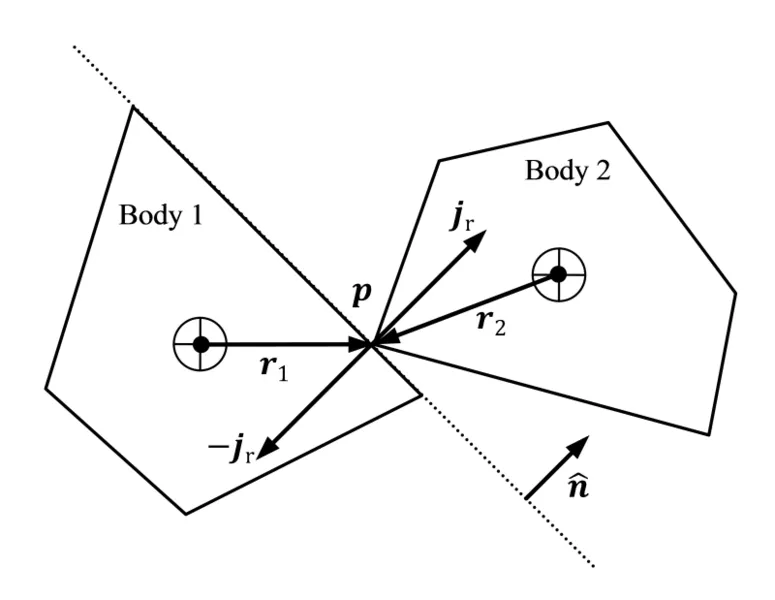[Image 1]

## Introduction

Hey it's a me again @drifter1! Today we continue with Physics and more specifically the branch "Classical Mechanics" to get into Impulse. So, without further ado, let's get straight into it!

## Impulse

In some of the previous articles of this series, we talked about Newton's second law and how the change in momentum is somewhat "hidden" inside of it. But, that is not the only quantity that we can derive from it. Until now we only saw that the change in momentum over time is equal to the applied force, and that alone is really useful in many calculations. What if we take the time and put it together with the force? Well, doing so we end up with:The quantity F • dt, which is equal to the change in momentum Δp, is called Impulse and symbolised using the capital letter J. The S.I. unit of Impulse is the Newton • Second (N • s). Being a vector quantity it goes in the same direction as the Force acting on the object. So, to summarize what we said until now, we can write: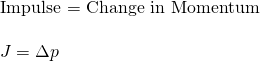Ok, so how is this new quantity useful? Well, impulse is very useful and important in real world applications where the force is not constant. It helps us analyze problems where the forces that act on an object's motion change over time. In the end, we don't simply multiply force with time, but find the area (integral) under a force-time curve. The overall net impulse will be equal to the total change in momentum.

So, even more generalized the so called Impulse-Momentum Theorem is: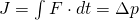But, note that all this can be applied to constant and "changing" mass, something that is applied in rocket propulsion physics! So, Impulse can be equal to: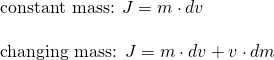For any given collision we can "play" with the Impact Force F and Collision Time t, keeping the Impulse and so momentum change the same, but reducing the overall "impact" from the collision.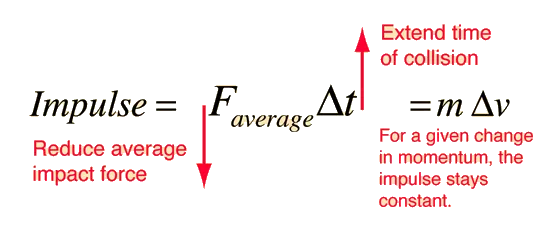[Image 2]

Similarly, the work required for any given change in kinetic energy stays constant, but we can reduce the impact force extending the collision distance: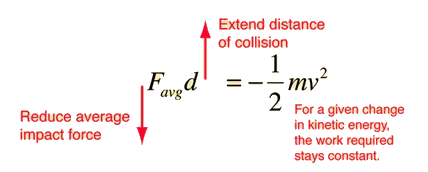[Image 3]

After using Newton's laws in so many equations, I guess that you can understand how Newton's laws are connected with momentum and impulse. More specifically we can write a table such as:

1st law Inertia
(m)
Momentum
(mv)
2nd law Force law
(ma)
Impulse-Momentum
(J = Δp)
3rd law Action-Reaction
(+F1 = -F2)
Conservation of Momentum
(Σp = Σp')

## Example from Sciencenotes

A 50 kg mass is sitting on a friction-less surface. An unknown constant force pushes the mass for 2 seconds until the mass reaches a velocity of 3 m/s. We have the following questions:
1. What is the initial momentum of the mass?
2. What is the final momentum of the mass?
3. What was the force acting on the mass?
4. What was the impulse acting on the mass?

### 1. Initial Momentum

Knowing that the object it at rest at first, the initial velocity is 0 m/s. Momentum is mass times velocity and so the initial momentum is: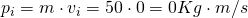### 2. Final Momentum

The final velocity of the object after the unknown force acts on it is 3m/s,., therefore the final momentum is: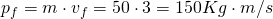### 3. Acting Force

Using Newton's second law, we can easily find the applied Force, as the change in momentum over time is equal to that Force. We know all the needed quantities, which are the change in momentum and the time that this change took place and so: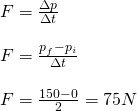### 4. Acting Impulse

The Impulse is equal to the constant Force F times the time that this force acted on the object. Therefore, the impulse is calculated to be: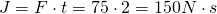This example was quite simple. Things can get more difficult when the forces that act are not constant and when more than one objects are affected by them. Either way, similarly to momentum-only problems, we have to look at the total system, as all these theorems apply to the whole system and rarely to single objects, in real world problems.

## RESOURCES:

### Images

1.2. http://hyperphysics.phy-astr.gsu.edu/hbase/impulse.html

## Final words | Next up

This is actually it for today's post! Next time we will get into the motion of the Center of Mass..

See ya!Keep on drifting!

H2
H3
H4
3 columns
2 columns
1 column